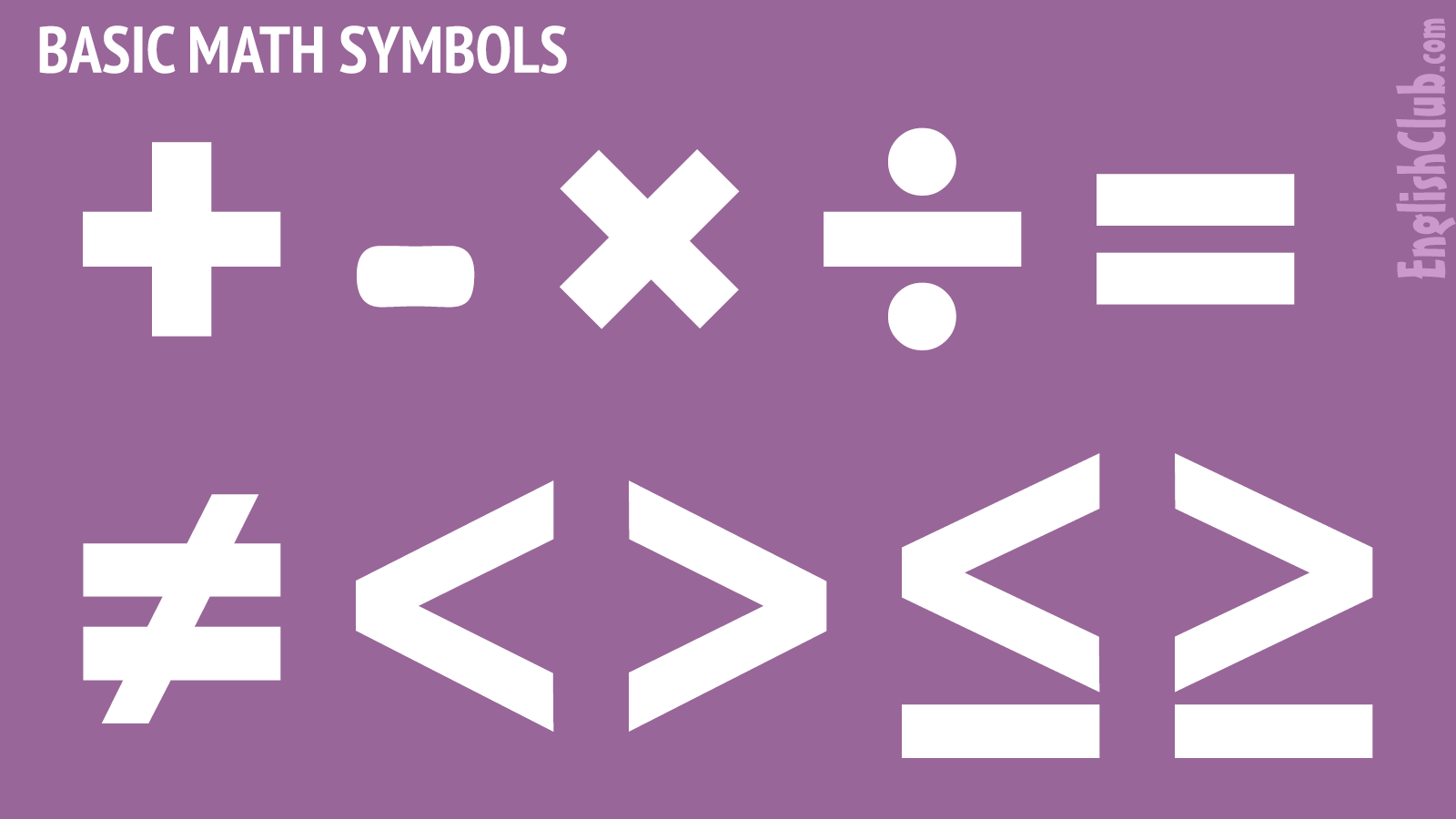Men go mad in herds and recover one by one

# Basic Maths Symbols

maths (BrE) | math (AmE) is the short form of mathematicsThis page lists basic maths symbols with their names and examples in English.

## +plus sign / addition sign

The plus sign represents:

### a. the notion of positive

Any number bigger than zero is a positive number and can be written with or without a plus sign in front of it.

Thus, +5 (plus five) and 5 (five) are the same number.

### b. the operation of addition

3 + 5 = 8
three plus five equals eight
five added to three makes eight
three added to five makes eight
if you add five to three you get eight

Addition gives us a sum. In 3 + 5 = 8, the sum is eight.

## -minus sign / subtraction sign

The minus sign represents:

### a. the notion of negative

Any number smaller than zero is a negative number and is written with a minus sign in front of it.

-3
minus three

### b. the operation of subtraction

8 - 5 = 3
eight minus five equals three
five subtracted from eight equals three
if you subtract five from eight you get three
if you take five from eight you get three

Subtraction gives us a difference. In 8 - 5 = 3, the difference is three.

## ×times sign / multiplication sign

The times sign represents:

### multiplication

5 x 6 = 30
five times six equals thirty
five multiplied by six equals thirty
five sixes are thirty
if you multiply 5 by 6 you get thirty

Multiplication gives us a product. In 5 x 6 = 30, the product is thirty.

## ÷ OR /division sign

The division sign represents:

### division

15 ÷ 3 = 5
15 / 3 = 5
fifteen divided by three equals five
five goes into fifteen three times
if you divide fifteen by three you get five
if you divide three into fifteen you get five

Division gives us a quotient. In 15 ÷ 3 = 5, the quotient is five.

Let us summarize the above four operations as:
operation   result
addition "plus" 2 + 2 = 4 sum
subtraction "minus" 5 - 3 = 2 difference
multiplication "times" 3 x 5 = 15 product
division "divided by" 21 / 7 = 3 quotient

## =equals sign

The equals sign represents equality:

3 + 4 = 7
three plus four equals seven

Note that we usually say equals NOT equal:

• two plus two equals four
• two plus two equal four

## <less than

3 < 4
three is less than four

## >greater than

4 > 3
four is greater than three

## ≠NOT equal to

x ≠ z
x is not equal to z

## ≥greater than or equal to

x ≥ z
x is greater than or equal to z

## ≤less than or equal to

z ≤ x
z is less than or equal to x

see fractions

## .decimal separator | point

The decimal separator separates a whole number from its fractional part to the right:

1.23

In English, the decimal separator is usually a point (.). Note that in some languages the decimal separator is a comma (,).

see decimals

## ,thousands separator

In English, the thousands separator separates whole numbers into groups of three from the right.

10,987,654,321

In English, the thousands separator is usually a comma (,). Note that in some languages the thousands separator is a point (.) or sometimes a space ( ).

see thousands

## %percent sign

The percent sign indicates a number or ratio as a fraction of 100 (percentage).

40%
forty percent
Only forty percent of the people voted for her.
What percentage voted for her? Forty percent.

## √square root

√16 = 4
the square root of sixteen equals four
the square root of sixteen is four

Nobody has the right to obey.'